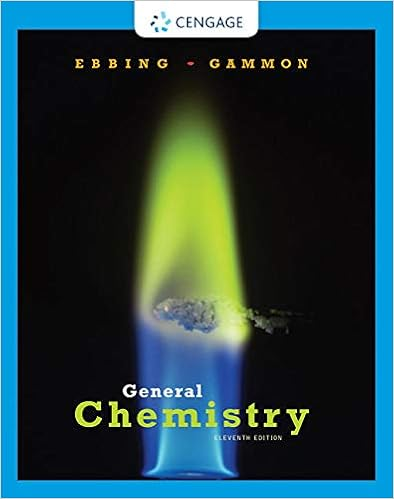# What is the ph of coffee if the h 3 o is 1 x 10 5 m 1

• Notes
• 59

This preview shows page 34 - 45 out of 59 pages.

##### We have textbook solutions for you!
The document you are viewing contains questions related to this textbook.The document you are viewing contains questions related to this textbook.
Chapter 15 / Exercise 15.5
General Chemistry
Ebbing/GammonExpert Verified
What is the pH of coffee if the [H 3 O + ] is 1 x 10 −5 M? 1) pH = 9.0 2) pH = 7.0 3) pH = 5.0
##### We have textbook solutions for you!
The document you are viewing contains questions related to this textbook.The document you are viewing contains questions related to this textbook.
Chapter 15 / Exercise 15.5
General Chemistry
Ebbing/GammonExpert Verified
Solution What is the pH of coffee if the [H 3 O + ] is 1 x 10 −5 M? STEP 1 Enter the [H 3 O + ] value STEP 2 Press log key and change the sign: log (1 x 10 −5 ) = [ 5] STEP 3 Make the number of digits after the decimal point (1) equal to the number of significant figures in the coefficient (1): [H 3 O + ]= 1 x 10 −5 , pH is 5. 0 (3)
[H 3 O + ], [OH - ], and pH Values
Neutralization is the reaction of an acid such as HCl and a base such as NaOH HCl( aq ) + H 2 O( l ) H 3 O + ( aq ) + Cl ( aq ) NaOH( aq ) Na + ( aq ) + OH ( aq ) the H 3 O + from the acid and the OH from the base to form water H 3 O + ( aq ) + OH ( aq ) 2H 2 O( l ) Neutralization Reactions
Acid–Base Titration Titration is a laboratory procedure used to determine the molarity of an acid uses a base such as NaOH to neutralize a measured volume of an acid Base (NaOH) Acid solution
Indicator An indicator is added to the acid in the flask causes the solution to change color when the acid is neutralized
End Point of Titration At the end point , the indicator has a light permanent color pink because all the acid is neutralized and the environment is now basic the volume of the base used to reach the end point is measured to determine the volume of NaOH used to reach the equivalence point , subtract the last drop of NaOH (0.05mL) the molarity of the acid is calculated using the neutralization equation for the reaction
End Point vs. Equivalence Point End point and equivalent point are not the same thing. The end point is what we can distinguish with our eyes, is when we see the indicator changing color The equivalence point is when: moles of acid = moles of base (exact neutralization) The indicator needs a basic environment to change color so when we see the change ( end point ) we are already in presence of excess base, we have passed the equivalence point Providing the user has been careful, and has recorded the end point as soon as the solution has turned LIGHT pink , the Volume of NaOH at equivalence point can be obtained subtracting one drop (0.05 mL) to the NaOH volume recorded at the end point
Equivalence Point of Titration At the equivalence point the number of moles of acid is equal to the number of moles of base: Moles acid (H + )= moles of base (OH - ) Since M= moles/V Then moles= M x V Therefore at the equivalence point (for reactions with 1:1 molar ratio of acid and base) M acid x V acid = M base x V base BUT… This is only true with monoprotic acids (one H + ) And monohydroxy bases (one OH - )
Titration problems Calculate the molarity of an HCl solution, if 15.00 mL of this HCl solution are completely neutralyzed *or titrated to the end point using 23.44 mL of a 0.135 M NaOH solution
We have seen that acids and bases react to form salt an water in neutralization reactions Neutralization is the reaction of an acid such as HCl and a base such as NaOH HCl( aq ) + NaOH( aq ) NaCl ( aq ) + H 2
•••